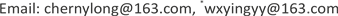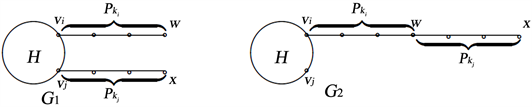﻿ 给定团数的Wiener指数极图 On the Extremal Wiener Indices of Graphs with Given Clique Number

Vol. 08  No. 06 ( 2019 ), Article ID: 31059 , 6 pages
10.12677/AAM.2019.86134

On the Extremal Wiener Indices of Graphs with Given Clique Number

Yuanlong Chen, Xiaoying Wu*

School of Financial Mathematics and Statistics, Guangdong University of Finance, Guangzhou GuangdongReceived: Jun. 11th, 2019; accepted: Jun. 21st, 2019; published: Jun. 28th, 2019ABSTRACT

In this paper, we investigate the Wiener index for connected graphs of given clique number, obtain sharp lower and upper bounds on Wiener index for connected graphs of order n with clique number l. For connected graphs of order n with clique number l, by the method of shift-joint deformation, we obtain the largest Wiener index graph and the smallest Wiener index graph are ${K}_{l}u{P}_{n-l+1}$ and Tuŕan graph, respectively.

Keywords:Graph, Wiener Index, Extremal Graphs, Clique Number1. 引言

Wiener指数可以用来解释烷烃的物理化学性质的变化，分子结构与药理性能的关系，因此确定Wiener指数的极值图对分子化学应用有着非常重要的意义。早在上个世纪70年代Entringer等在  中证明了n阶树中具有最大、最小Wiener指数的极值图分别是路 ${P}_{n}$ 与星图 ${S}_{n}$ 。随后出现许多关于Wiener指数的数学与化学的结论，特别是树的Wiener指数研究。例如Jelena等在  中讨论了给定最大度的n阶树，获得此类树的最小Wiener指数极值图。刘慧清等在   中分别讨论了给定直径的n阶树的最小Wiener指数极值图与及给定r个圈的n阶连通图的四类拓扑指数极值图。王华在研究给定度序列的n阶树时  ，确定了最大与最小的Wiener指数极值图。Dobrynin等在  中对近年关树的Wiener指数的重要研究结果做了一个综述，并给出了几个猜想及公开问题。

2. 基本引理

$\begin{array}{l}H\left({K}_{l},T\right)=\left\{H\left({K}_{l};{T}_{{k}_{1}},{T}_{{k}_{2}},\cdots ,{T}_{{k}_{l}}\right):n=\underset{i=1}{\overset{l}{\sum }}{k}_{i},{k}_{i}\ge 1,i=1,2,\cdots ,l\right\}\\ H\left({K}_{l},P\right)=\left\{H\left({K}_{l};{P}_{{k}_{1}},{P}_{{k}_{2}},\cdots ,{T}_{{k}_{l}}\right):n=\underset{i=1}{\overset{l}{\sum }}{k}_{i},{k}_{i}\ge 1,i=1,2,\cdots ,l\right\}\end{array}$

${D}_{{P}_{n}}\left(v\right)={D}_{{P}_{k}}\left(u\right)+{D}_{{P}_{n}\{P}_{k}}\left(u\right)\ge {D}_{{P}_{k}}\left(u\right)+k\left(n-k\right)$

$V\left(G\right)=V\left({H}_{1}\right)\cup V\left({H}_{2}\right)$$V\left({H}_{1}\right)\cap V\left({H}_{2}\right)=\left\{v\right\}$$E\left(G\right)=E\left({H}_{1}\right)\cup E\left({H}_{2}\right)$

$H={G}_{1}\\left(\left({P}_{{k}_{i}}-\left\{{v}_{i}\right\}\right)\cup \left({P}_{{k}_{j}}-\left\{{v}_{j}\right\}\right)\right),{G}_{2}=H{v}_{i}{P}_{{k}_{i}}w{P}_{{k}_{j}}$ (见图1)。则 $W\left({G}_{2}\right)>W\left({G}_{1}\right)$Figure 1. Two graphs

$W\left({G}_{1}\right)=W\left({P}_{{k}_{i}}{v}_{i}H\right)+W\left({P}_{{k}_{j}}\right)+\left({n}_{{P}_{{k}_{i}}{v}_{i}H}-1\right){D}_{{P}_{{k}_{j}}}\left({v}_{j}\right)+\left({k}_{j}-1\right){D}_{{P}_{{k}_{i}}{v}_{i}H}\left({v}_{j}\right)$

$W\left({G}_{2}\right)=W\left(H{v}_{i}{P}_{{k}_{i}}\right)+W\left({P}_{{k}_{j}}\right)+\left({n}_{H{v}_{i}{P}_{{k}_{i}}}-1\right){D}_{{P}_{{k}_{j}}}\left(w\right)+\left({k}_{j}-1\right){D}_{H{v}_{i}{P}_{{k}_{i}}}\left(w\right)$

$\begin{array}{c}{D}_{H{v}_{i}{P}_{{k}_{i}}}\left(w\right)={D}_{H\\left\{{v}_{i}\right\}}\left(w\right)+{D}_{{P}_{{k}_{i}}}\left(w\right)\\ ={D}_{H\\left\{{v}_{i}\right\}}\left({v}_{i}\right)+\left({k}_{i}-1\right)\left({n}_{H}-1\right)+{D}_{{P}_{{k}_{i}}}\left({v}_{i}\right)\\ ={D}_{H\\left\{{v}_{i}\right\}}\left({v}_{i}\right)+\left({k}_{i}-1\right)\left({n}_{H}-1\right)+{D}_{{P}_{{}_{{k}_{i}}}}\left({v}_{j}\right)-{k}_{i}\\ ={D}_{H\\left\{{v}_{i},{v}_{j}\right\}}\left({v}_{j}\right)+{d}_{H\left({v}_{i},{v}_{j}\right)}+\left({k}_{i}-1\right)\left({n}_{H}-1\right)+{D}_{{P}_{{}_{{k}_{i}}}}\left({v}_{j}\right)-{k}_{i}\\ ={D}_{H\\left\{{v}_{i}\right\}}\left({v}_{j}\right)+1+\left({k}_{i}-1\right)\left({n}_{H}-1\right)+{D}_{{P}_{{}_{{k}_{i}}}}\left({v}_{j}\right)-{k}_{i}\\ ={D}_{H\\left\{{v}_{i}\right\}}\left({v}_{j}\right)+\left({k}_{i}-1\right)\left({n}_{H}-\text{2}\right)+{D}_{{P}_{{}_{{k}_{i}}}}\left(vj\right)\end{array}$

${D}_{H{v}_{i}{P}_{{k}_{i}}}\left({v}_{j}\right)={D}_{H\\left\{{v}_{i}\right\}}\left({v}_{j}\right)+{D}_{{P}_{{k}_{i}}}\left(vj\right)$

$W\left({G}_{2}\right)-W\left({G}_{1}\right)=\left({n}_{H{v}_{i}{P}_{{k}_{i}}}-1\right){D}_{{P}_{{k}_{j}}}\left({v}_{j}\right)+\left({k}_{j}-1\right)\left({D}_{H{v}_{i}{P}_{{k}_{i}}}\left(w\right)-{D}_{{P}_{{k}_{i}}{v}_{i}H}\left({v}_{j}\right)\right)>0$

$W\left({G}_{2}\right)>W\left({G}_{1}\right)$

3. 项给定团数的图的Wiener指数极图

$W\left(G\right)\ge \left(\begin{array}{c}n\\ 2\end{array}\right)+n-l+n-l-{r}_{1}>\left(\begin{array}{c}n\\ 2\end{array}\right)+n-l=W\left({T}_{l,n}\right)$

$\begin{array}{c}W\left(G\right)\ge \left(\begin{array}{c}n\\ 2\end{array}\right)+n-l+n-l-{r}_{1}+\cdots +n-l-\underset{i=1}{\overset{k-1}{\sum }}{r}_{i}\\ >\left(\begin{array}{c}n\\ 2\end{array}\right)+n-l+\cdots +n-⌊\frac{n}{l}⌋l=W\left({T}_{l,n}\right)\end{array}$

$n=tl+r\left(0\le r ，则 $t=⌊\frac{n}{l}⌋$ 。由断言3可知G中有t个顶点互不相交的l团，不妨设为 ${K}_{{l}_{1}},{K}_{{l}_{2}},\cdots ,{K}_{{l}_{t}}$$V\left({K}_{{l}_{1}}\right)=\left\{{u}_{1},{u}_{2},\cdots ,{u}_{l}\right\}$$V\left({K}_{{l}_{2}}\right)=\left\{{u}_{l+1},{u}_{l+2},\cdots ,{u}_{2l}\right\}$ ，…，

$V\left({K}_{{l}_{t}}\right)=\left\{{u}_{\left(t-1\right)l+1},{u}_{\left(t-1\right)l+2},\cdots ,{u}_{tl}\right\}$

$V\left({K}_{r}\right)=\left\{{u}_{tl+1},{u}_{tl+2},\cdots ,{u}_{tl+r-1},{u}_{n}\right\}$ 。下面说明这些团之间哪些顶点相邻。

(i) 对前t个l团中任意两个l团必有其中一个团的每个顶点正好与另一个团的 $l-1$ 个顶点都相邻.根据G的团数为l，则其中任意一个团的每个顶点至多与另一个团的 $l-1$ 个顶点都相邻。否则，G中存在阶数为 $l+1$ 的团，这与G的团数为l矛盾。根据 $W\left(G\right)$ 尽可能小及断言3中类似分析可知，其中任意一个团的每个顶点正好与另一个团的 $l-1$ 个顶点都相邻。

$\begin{array}{l}{u}_{kl+i}{u}_{sl+i}\notin E\left(G\right)\left(\text{1}\le i\le l,0\le k\ne s\le t-1\right),\\ {u}_{kl+i}{u}_{sl+j}\in E\left(G\right)\left(\text{1}\le i\ne j\le l,0\le k\ne s\le t-1\right),\end{array}$

(ii) 类似上面的讨论，可知第 $t+1$ 个r团 ${K}_{r}$ 中每个顶点正好与前t个顶点互不相交的l团 ${K}_{{l}_{i}}\left(1\le i\le t\right)$

$l-1$ 个顶点都相邻。不失一般性可设

$\begin{array}{l}{u}_{tl+i}{u}_{sl+i}\notin E\left(G\right)\left(1\le i\le r,0\le s\le t-1\right),\\ {u}_{tl+i}{u}_{sl+j}\in E\left(G\right)\left(i\ne j,1\le i\le r,1\le j\le l,0\le s\le t-1\right),\end{array}$

$\left(j=r+1,r+2,\cdots ,l\right)$ 构成 $l-r$ 个顶点数为t的空图 ${\stackrel{¯}{K}}_{t}$

On the Extremal Wiener Indices of Graphs with Given Clique Number[J]. 应用数学进展, 2019, 08(06): 1160-1165. https://doi.org/10.12677/AAM.2019.86134

1. 1. Entringer, R. and Jackson, D. (1976) Snyder D Distance in Graphs. Czechoslovak Mathematical Journal, 26, 283-296.

2. 2. You, Z.-F., Huang, Y. and Du, X. (2018) A Note on Comparison between the Wiener Index and the Za-greb Indices. Communications in Mathematical Research, 34, 296-302.

3. 3. Das, K.C. and Nadjafi-Arani, M.J. (2017) On Maximum Wiener Index of Trees and Graphs with Given Radius. Journal of Combinatorial Optimization, 34, 574-587. https://doi.org/10.1007/s10878-016-0092-y

4. 4. Gutman, I. and Yeh, Y.N. (1995) The Sum of All Dis-tances in Bipartite Graphs. Mathematica Slovaca, 45, 327-334.

5. 5. Berega, S. and Wang, H. (2007) Wiener Indices of Balanced Binary Trees. Discrete Applied Mathematics, 155, 457-467. https://doi.org/10.1016/j.dam.2006.08.003

6. 6. Czabarkaě, E., Szěkely, L. and Wagner, S. (2009) The Inverse Problem for Certain Tree Parameters. Discrete Applied Mathematics, 157, 3314-3319. https://doi.org/10.1016/j.dam.2009.07.004

7. 7. Li, X.L. and Wang, L. (2004) Solutions for Two Conjectures on the Inverse Problem of the Wiener Index of Peptoids. SIAM Journal on Discrete Mathematics, 17, 210-218. https://doi.org/10.1137/S0895480101387261

8. 8. Wu, X.Y. and Liu, H.Q. (2010) On the Wiener Index of Graphs. Acta Applicandae Mathematicae, 110, 535-544. https://doi.org/10.1007/s10440-009-9460-2

9. 9. Liu, H.Q. and Pan, X.F. (2008) Minimal Wiener Index of Trees with Fixed Diameter. MATCH Communications in Mathematical and in Computer Chemistry, 60, 85-94.

10. 10. Dimitrov, D., Ikica, B. and Škrekovski, R. (2019) Maximum External Wiener Index of Graphs. Discrete Applied Mathematics, 257, 331-337. https://doi.org/10.1016/j.dam.2018.09.024

11. 11. Lepovic, M. and Gutman, I. (1998) A Collective Property of Trees and Chemical Trees. Journal of Chemical Information and Modeling, 38, 823-826. https://doi.org/10.1021/ci980004b

12. 12. Goldman, D., Istrail, S., Lancia, G., Piccolboni, A. and Walenz, B. (2000) Algorithmic Strategies in Combinatorial Chemistry. In: Proceedings of the 11th Annual ACM-SIAM Symposium on Discrete Algorithms, Society for Industrial and Applied Mathematics, Philadelphia, PA, 275-284.

13. 13. Wagner, S. (2006) A Class of Trees and Its Wiener Index. Acta Applicandae Mathematicae, 91, 119-132. https://doi.org/10.1007/s10440-006-9026-5

14. 14. Ban, Y.-E.A., Bespamyatnikh, S. and Mustafa, N.H. (2004) A Conjecture on Wiener Indices in Combinatorial Chemistry. Algorithmica, 40, 99-117. https://doi.org/10.1007/s00453-004-1097-y

15. 15. Wang, H. (2008) The Extremal Values of the Wiener Index of a Tree with Given Degree Sequence. Discrete Applied Mathematics, 156, 2647-2654. https://doi.org/10.1016/j.dam.2007.11.005

16. NOTES

*通讯作者。